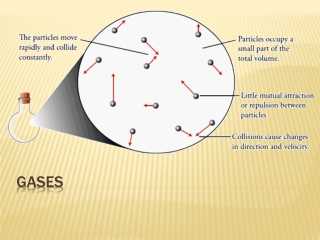DownloadDownload PresentationGases

# Gases

Télécharger la présentation## Gases

- - - - - - - - - - - - - - - - - - - - - - - - - - - E N D - - - - - - - - - - - - - - - - - - - - - - - - - - -
##### Presentation Transcript

1. Gases

2. Properties of Gases • Gases uniformly fill any container • Gases are easily compressed • Gases mix completely with any other gas • Gases exert pressure on their surroundings Pressure

3. Measuring barometric pressure • The barometer • Invented by Evangelista Torricelli in 1643 • Units • mm Hg (torr) 760 torr = 1 atm • newtons/m2 (pascal (Pa)) 101,325 Pa = 1 atm 101,325 Pa = 101.3 kPa • Atmospheres 1 atm = standard pressure Pressure

4. Boyle’s law (Robert Boyle) The product of pressure times volume is a constant, provided the temperature and number of moles remains the same • Pressure and volume are inversely related • Volume increases linearly as the pressure decreases (1/P) The Gas Laws of Boyle, Charles and Avogadro

5. Boyle’s Law Continued…… • At constant temperature, Boyle’s Law can be used to find a new volume or pressure • Boyle’s law works best at low pressures • Gases that obey Boyle’s Law are called Ideal Gases The Gas Laws of Boyle, Charles and Avogadro

6. Charles’ Law (Jacques Charles) The volume of a gas increases linearly with temperature provided the pressure and number of moles remain constant. Temperature and volume are directly proportional The Gas Laws of Boyle, Charles and Avogadro

7. Charles’ Law continued……….. • Temperature must be measured in degrees Kelvin • K = oC+ 273 • 0 K is “absolute zero” The Gas Laws of Boyle, Charles and Avogadro

8. Avogadro’s Law (Amedeo Avogadro) For a gas at constant temperature and pressure, the volume is directly proportional to the number of moles. The Gas Laws of Boyle, Charles and Avogadro

9. Combined gas law – (n remaining constant) The Combined Gas Law

10. Derived from existing laws……. • V = k/P, V = bT and V = an • V = (k)(b)(a)(Tn/P) • Constants k, b and a are combined into the universal gas constant R and….. The Ideal Gas Law

11. Limitations of the Ideal Gas Law • Works well at low pressure and high temperatures • Most gases do not behave ideally above 1 atm • Does not work well near the condensation conditions of a gas The Ideal Gas Law

12. Variations of the Ideal Gas Law DensityMolar Mass of a gas The Ideal Gas Law

13. “For a mixture of gases in a container, the total pressure exerted is the sum of the pressures each gas would exert if it were alone.” It is the total number of moles that is important, not the identity or composition of the gas particles. Dalton’s Law of Partial Pressure

14. At Standard Temperature and Pressure (STP) T = 273.15 K (0oC) P = 1 atm (760 torr or 101.3 kPa) 1 mole of an ideal gas occupies 22.4 L of volume Remember…….. Density = mass/volume and moles (n)= grams/molar mass gas stoichiometry